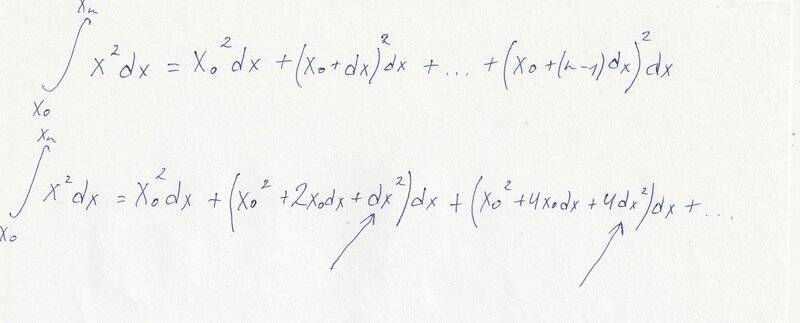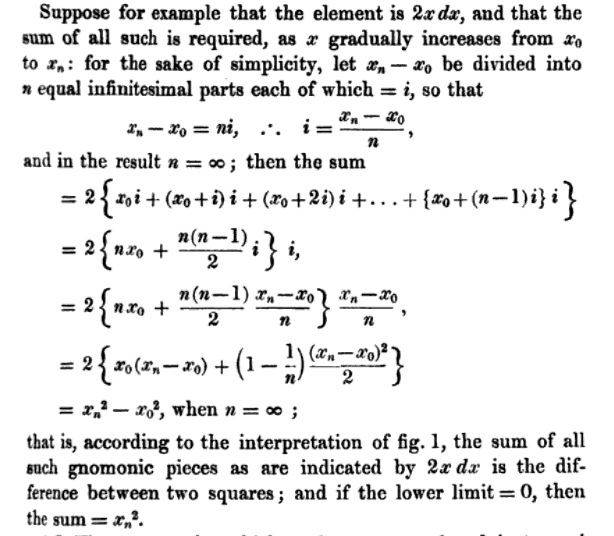# Neglected terms in integral sum

• B
• Mike_bb
The author's use of dx in the Riemann sum is not at all the way things are usually done these days. See https://en.wikipedia.org/wiki/Riemann_sum for a more modern explanation.f

#### Mike_bb

Hello.

As is known, we can neglect high-order term in expression ##f(x+dx)-f(x)##. For ##y=x^2##: ##dy=2xdx+dx^2##, ##dy=2xdx##.

I read that infinitesimals have property: ##dx+dx^2=dx##

I tried to neglect high-order terms in integral sum (##dx^2## and ##4dx^2## and so on) and I obtained wrong result in the end.Where is my mistake?

Thanks.

Hello.

As is known, we can neglect high-order term in expression ##f(x+dx)-f(x)##. For ##y=x^2##: ##dy=2xdx+dx^2##, ##dy=2xdx##.

I read that infinitesimals have property: ##dx+dx^2=dx##

I tried to neglect high-order terms in integral sum (##dx^2## and ##4dx^2## and so on) and I obtained wrong result in the end.

View attachment 304873

Where is my mistake?

Thanks.
I don't really understand what you've done. The ##dx## in an integral isn't an infinitesimal.

In fact, to be precise, there is no such thing as an "infinitesimal" in standard calculus. Although, there is the concept of the "differential" ##dx##.

The ##dx## in the integral is not a differential either, but a notation specific to integration.

I don't really understand what you've done. The ##dx## in an integral isn't an infinitesimal.

In fact, to be precise, there is no such thing as an "infinitesimal" in standard calculus. Although, there is the concept of the "differential" ##dx##.

The ##dx## in the integral is not a differential either, but a notation specific to integration.
I read "A treatise on infinitesimal calculus" Bartholomew Price. Integral sum, for ##2xdx##:•PeroK
Okay. Infinitesimal calculus is not something I know anything about, I'm sorry to say!

•Mike_bb
@Mike_bb, what you're doing is evaluating the definite integral using a Riemann sum.
In post 1 you said this:
Mike_bb said:
For ##y = x^2, dy = 2xdx + dx^2, dy = 2xdx##
This doesn't make any sense to me. If the function is defined by the formula ##y = f(x) = x^2##, then by definition, the differential of f (df) or differential of y (dy) is given by ##dy = \frac{df}{dx} dx = 2xdx##. I don't see where you're getting the ##dx^2## term from.

In evaluating the def. integral ##\int_{x_0}^{x_n} x^2 dx##, the author of the book you're working from is using a Riemann sum to evaluate the integral. BTW, from the language used, my guess is that this is a very old textbook (maybe over 100 years old!). I would advise getting something a bit more modern and that uses illustrations.

The author's use of dx in the Riemann sum is not at all the way things are usually done these days. See https://en.wikipedia.org/wiki/Riemann_sum for a more modern explanation.

In the Riemann sum, the interval ##[x_0, x_n}## is divided into subintervals of equal length. This is usually written as ##\Delta x = \frac{x_n - x_0} n## and not as dx.

In each subinterval, an estimate is calculated of the area under the curve, within that subinterval, and then all n subintervals are added to get the total. This is easier to follow from a drawing, several of which are shown with examples in the wiki article I linked to.

•PeroK